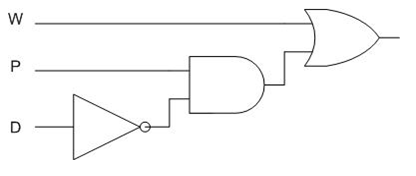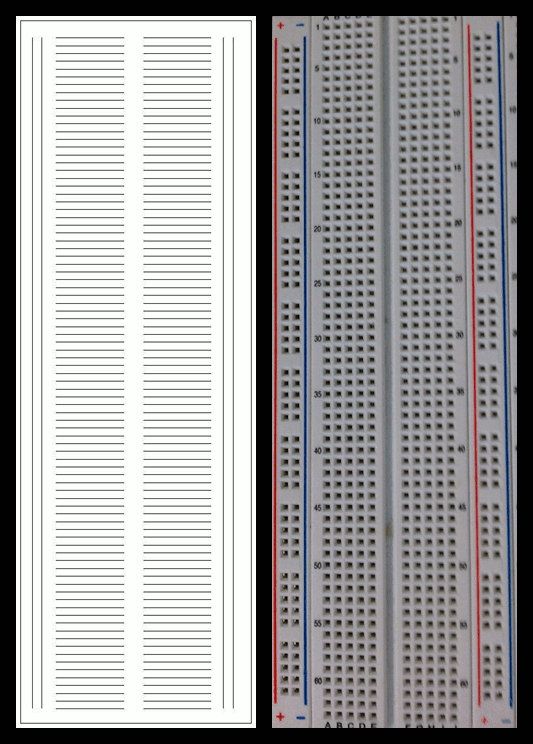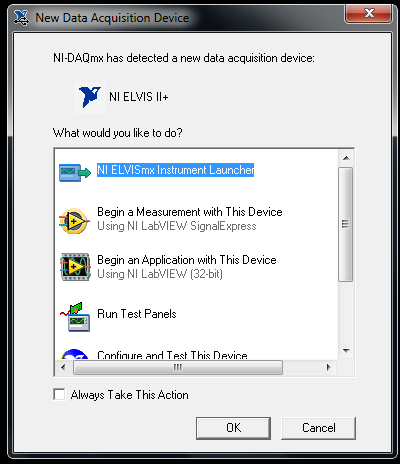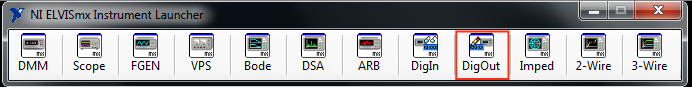NI ELVIS Tutorial Video

General Engineering has created a video that discusses breadboard wiring and digital logic circuits: NI ELVIS Tutorial Video. This video provides information on the educational NI-ELVIS II + prototyping board that will aid in designing and wiring the physical circuit. Material covered in the video will be on the Lab 7 quiz. Viewing the video is a mandatory pre-lab assignment.

Objectives

The experimental objective of this lab is to design a combinational logic circuit that will activate under specific conditions and test it using LabVIEW. After testing, it will be built on an NI-ELVIS II+ prototyping board.

Overview

The first step in understanding the digital circuits that control the function of electronic devices is the mastery of Boolean logic. George Boole, an English mathematician, established modern symbolic logic in 1854 with the publication of his paper, "Laws of Thought." Boolean logic is the foundation of digital circuitry. Boole's method of logical inference allows conclusions to be drawn from any proposition involving any number of terms. Boolean logic demonstrates that the conclusions found are logically contained in the original premises.

In Boolean logic, there are only two values, true and false, represented by the numbers 1 and 0, respectively. These values are combined in equations to yield results that also have these two values. The equations are represented by truth tables that show the inputs to the equation and the outputs produced for those inputs. The rows of the table contain all the possible combinations of 1s and 0s for the inputs. The number of rows is determined by the number of possible combinations.

Boolean logic is applied to digital circuitry through the use of simple logic gates. There are symbols for each of these gates, and the connections between them are represented by lines running from the output of one gate to the input of the other. A line can connect only one output to each input. There are seven of these gates: the NOT, AND, OR, NAND, NOR, XOR, and XNOR gates. Only the first three will be used in this lab.

The NOT gate is the simplest of these three. It is an inverter. It has one input and produces its opposite as output. The symbol for a NOT gate is shown in Figure 1.

The truth table for a NOT gate has one input, or A in this example, and one output. The symbol for the operation is a horizontal bar over the variable. The truth table for a NOT gate is shown in Table 1.0 1
1 0

The AND gate performs a multiplication operation on its inputs. If all the inputs are true, the output is also true. But if either of the inputs is false, the output is also false. An AND gate can have two or more inputs, but for this lab, it will have two inputs. The symbol for an AND gate is shown in Figure 2.

This gate has two inputs, or A and B in this example, and one output. The symbol for the AND operation is a dot (·) or the two inputs one after the other with nothing between them. The truth table for an AND gate is shown in Table 2.0 0 0
0 1 0
1 0 0
1 1 1

An OR gate performs an addition operation on its inputs. If either of the inputs is true, the output is also true. But if all the inputs are false, the output is also false. An OR gate can have two or more inputs, but for this lab, it will have two inputs. The symbol for an OR gate is shown in Figure 3.

An OR gate has two inputs, or A and B in this example, and one output. The symbol for the OR operation is a plus (+). The truth table for an OR gate is shown in Table 3.0 0 0
0 1 1
1 0 1
1 1 1

The truth table attached to each of these gates indicates when the gate will return a value of true. These tables are used to write a Boolean equation for a problem. All the combinations that yield an output of 1 are kept, and the equation is written. This is called a Sum of Products solution.

A truth table showing every possible combination of inputs and their corresponding outputs yields a Boolean equation that is the solution to a problem in digital logic. This equation can be simplified using a Karnaugh map (K-map). A K-map identifies and eliminates all the conditions that do not contribute to the solution. This simplified equation is used to build the digital circuit and will be a combination of the gates described above.

A K-map is a two-dimensional representation of the truth table that shows the common characteristics of the inputs. For an equation with three inputs, usually all the combinations of the first two inputs are shown as four columns and the values for the third input are shown as two rows. For four inputs, all the combinations of the third and fourth inputs are shown as four rows. Only one value can change at a time in adjacent rows or columns. For example, in the truth table in Table 4, A, B, and C are the inputs and D is the output.0 0 0 0
0 0 1 1
0 1 0 0
0 1 1 1
1 0 0 0
1 0 1 1
1 1 0 1
1 1 1 1

This table looks complex, but it can be simplified with a K-map (Table 5).

0 0 0 1 1 1 1 000 0 1 0
11 1 1 1

Look for the cells that have values in common. For example, the entire bottom row has ones in all its cells. This means that any time C is true, the output is true. This can be done in a more organized manner. Look for cells that form rectangles, where the number of cells in the rectangle is a power of 2: 1, 2, 4, or 8 and so on. The bottom row is an example of this. There is another small rectangle of two cells in the column with 1 1 at the top. This means that the output is true whenever A and B are true. The rectangles are allowed to overlap so the same cells can be used more than once, which happened here. Look for the biggest rectangles first, and keep finding smaller rectangles until all the cells with a 1 in them are used. This means that sometimes just single cells will remain at the end. In this example, all the cells containing a 1 were covered with two rectangles. This means is that if the conditions for either rectangle are true, the output will be true. Looking at the row and column labels, the bottom row is C, and for the column, the header is AB. This yields the simplified Boolean equation shown in Figure 4.Figure 4: Simplified Boolean Equation

This equation meets the conditions in the truth table and achieves the desired result with the minimum number of logic elements.

Materials and Equipment

• A 7432 IC (4 dual-input OR gates)
• A 7408 IC (4 dual-input AND gates)
• A 7404 IC (6 single input NOT gates)
• A lab PC with LabVIEW software
• An NI-ELVIS II+ with prototyping board
• Wiring

Sample Problem

Boolean Equation

The combinations that yield an output of 1 are kept. For each of these combinations, determine the input values and multiply those variables together. If an input is true, it can be used as is; for an input that is false, invert it using the horizontal bar NOT operation. Since the output is true if any of the combinations are true, the equation is formed by using addition operations on all the terms formed by the product of each combination. The Boolean equation in Figure 5 is created.Figure 5: Boolean Equation

Karnaugh Map

A table like the one in Table 7 that maps out all the possible combinations is drawn. Only one variable can change at a time between adjacent columns. For this example, the columns will change from 00 to 01 to 11 to 10, as shown in Table 7. A TA will explain this further. The cells that are true are grouped in rectangles of two, four, or eight cells. This shows the values that these cells have in common. These common values yield the simplified Boolean equation. The K-map in Table 7 corresponds to the Boolean equation created.

Compare this K-map in Table 7 with the equation to see why some cells are 1 and the others are 0.

Table 7: K-map.
0 0 0 1 1 1 1 000 1 1 1
10 1 1 0

Simplified Boolean Equation

Form the largest rectangles of two, four, and eight cells. There is a rectangle of four cells (2 rows by 2 columns) plus an overlapping rectangle of two cells (1 row by 2 columns), as shown in Table 8.

Always try to group the greatest number of common values in powers of 2.

To create a simplified Boolean equation, group all the combinations together and eliminate those that contain both values of a variable. For example, in the big rectangle in the middle, W is always true, but the rectangle also includes both the true and false values of P and D. This means that if W is true, the values of P and D do not matter since all their combinations are included. Similarly, for the small rectangle, if P is true and D is false, the value of W does not matter since both its true and false values are included in the rectangle. Putting this together, if W is true, or P is true and D is false, the output should be true, and this includes all the input conditions required to produce a true output. A simplified Boolean equation for this problem is shown in Figure 6.Figure 6: Simplified Boolean equation

This equation in Figure 6 is much simpler than the original equation, but is fully equivalent to it.

Combinational Logic Circuit

Construct a combinational logic circuit using the simplified equation. If a NOT operation is performed on D, and an AND operation is performed on the result then P, the second term in the equation, is found. This result combined with performing an OR operation with W produces the result.

This is shown in Figure 7.Figure 7: Combinational logic circuit diagram for the simplified equation.

Problem Statement

A farmer owns a 350-acre dairy farm in upstate New York. In addition to milk and butter, the farmer sells fresh eggs at the Union Square Greenmarket in Manhattan. It is imperative that the farmer protect the hen that lays his eggs.

He has two barns, one hen, and a supply of corn. A fox has been attempting to eat the hen by hiding in a barn. The hen can move freely from one barn to the other. Farmer Georgi sometimes stores corn in one barn and sometimes in the other, but he never stores it in both at the same time. The hen would like to eat the corn and the fox would like to eat the hen. Farmer Georgi needs an alarm system that uses digital logic circuits to protect the hen and the corn. The design should use the fewest gates and input variables possible. The alarm will sound if:

1. The fox and the hen are in the same barn
2. The hen and the corn are in the same barn

Procedure

Prior to Beginning the Procedure

Before performing the lab, the integrated circuits (IC) used must be tested to determine if they are functioning. If the procedure is completed without verifying that the ICs work, problems may occur in the circuit that require additional time and troubleshooting. The system for checking the ICs uses an Arduino to send and receive signals from the AND/OR/NOT chips and determines if they are functioning properly.

First, load the chip onto the IC tester (Figure 8) and start the Serial Monitor on the Arduino program. The code will cycle through the basic inputs (0, 1 for NOT; 00, 01, 10, 11 for AND/OR) and then send the outputs of each gate within the chip back into the Arduino. The code compares those results to the expected result. It displays each input/output pair and displays the final result showing if the chip is functional or defective. The goal is to determine if the gates are outputting the correct results. Remove the chip carefully from the IC tester.

Problem Statement Procedure

Note: The two barns must be assigned a numeric equivalent before the truth table is prepared for Step 1. Use 1 for Barn 1 and 0 for Barn 2. For the alarm output, use 0 to indicate the alarm should be off, and 1 to indicate that the alarm should be on.

1. On a sheet of lined lab note paper from the EG Website, create a truth table that includes three inputs and one output. Assign the input variables. Include all possible scenarios for the hen, the corn, and the fox.
2. Compute the output column. To do this, analyze the three inputs and determine if the alarm will sound in each scenario. Place a 1 in the output column if the alarm will sound and a 0 if it will not.
3. Note all the combinations that produce a 1 in the output column.
4. Create a Boolean equation from this table that includes each of the inputs that produced a true output.
5. Create a K-map. Draw a map that lists all the possible combinations. Use the Boolean equation to fill in the 1s on the K-map.
6. Circle the pairs of 1s. The 1s may only be circled in powers of 2 starting with the largest possible combination and working down to the smallest. The outcomes that do not contribute to the solution are discarded.
7. This process yields a simplified Boolean equation. Write this simplified equation down.
8. Draw a schematic diagram of the simplified Boolean equation.
9. Have a TA sign the diagram.
10. Test the circuit diagram. Build the circuit in LabVIEW. Use the simplified Boolean equation. Open LabVIEW and select New VI. Pull down the Window menu, select Tile Left and Right.
11. Place three switches on the front panel that represent the hen, the corn, and the fox. Place one Boolean indicator on the front panel to represent the output.
12. Open the Functions palette. Under Programming, select Boolean and choose the AND, NOT, and OR gates necessary for the circuit. A TA will assist in this process.
13. Once the LabVIEW program is complete, have a TA check the circuit.
14. Build the circuit on a prototyping board.
15. On the board, identify each of the three black chips as an AND, OR, or NOT gate. To do this, read the number on the chip and match it with the list in the Materials and Equipment section of this lab. Use the pinouts in Figure 9 to wire the gates. Look for the notch at one end of each chip to orient the chip to diagrams in Figure 9 to match the pinouts.
16. Power the chips. Insert one end of the wire into a hole on Row 54 (marked +5V) of the side breadboards. Insert the other end of the wire into the hole on the breadboard nearest Pin 14 on the chip. Repeat this process for all three chips.Figure 10: Breadboard layout. Wiring diagram, left. Photograph, right.
The breadboard is set up in the following manner:
1. The leftmost and rightmost columns of slots (the ones in between the red and blue lines) are connected vertically.
2. The rest of the breadboard is connected horizontally, however, there is a break that runs down the center of the breadboard, separating Columns A-E and F-J, as shown in Figure 10.
17. Ground the chips using the same method. Insert one end of a wire into a hole on Row 53 (marked Ground) of the small breadboards and the other end into Pin 7 of the chip. Repeat this process for all three chips.
18. Look at the top right corner of the breadboard to find the Digital Input/Output (DIO) holes. Select one of the DIO row of holes to use as one of the variables and begin wiring the circuit based on the simplified Boolean equation. Assign the other DIO rows for the remaining variables.
19. Continue this process until the entire circuit is wired. Insert one end of the wire into the final output of the simplified Boolean equation. Attach the other end to any hole marked LED on the board.
20. Plug in the NI-ELVIS II+ and connect the USB cable to the lab PC.
21. Power on the NI-ELVIS II+ by toggling the switch on the back of the device. When the pop-up window appears, select NI-ELVISmx Instrument Launcher and click OK (Figure 11).Figure 11: New data acquisition device dialog window.
22. Click on the DigOut Icon on the Instrument Launcher window (Figure 12).Figure 12: NI ELVISmx Instrument Launcher dialog window.
23. Select the appropriate range for the Lines to Write setting in the Digital Writer dialog window that corresponds to the DIO rows chosen. For example, if fox, hen, and corn are on DIO 21, DIO 22, and DIO 23, select 16–23.
24. Click Run and toggle the switches corresponding to fox, hen, and corn.
25. Have a TA check the circuit.

The lab work is now complete. Please clean up the workstation. Return all unused materials to a TA. Refer to Assignment for the instructions to prepare the lab report.

Assignment

Individual Lab Report

Follow the lab report guidelines laid out in the page called Specifications for Writing Your Lab Reports in the Technical Communication section of this manual. The following points should be addressed in the appropriate section of the lab report:

• Describe how digital logic impacts the real world
• Describe the problem that was solved in the Introduction
• Describe how AND, OR, and NOT gates work
• What is a truth table? How does it contribute to obtaining a Boolean equation?
• What is a K-map? How does it contribute to obtaining a simplified Boolean equation? To demonstrate the simplification, show what the equation would look like if terms were written for each of the true values and the simplified equation by applying the K-map. Discuss how much simpler the circuitry is as a result of applying the K-map
• Describe how to construct a digital logic circuit using Boolean equations and K-maps
• Explain why the alarm system did or did not work
• Why is there a need for the minimization of a logic design and what are the advantages?
• How does the use of a combinational logic circuit contribute to advances in technology?
• Describe the design changes that would be necessary if one of the barns used a bell as an alarm and the other used a horn
• Describe how the final combinational logic circuit was obtained
• Insert a screenshot of the front and back panels in the report

Remember: Lab notes must be taken. Experimental details are easily forgotten unless written down. EG1003 Lab Notes Paper can be downloaded and printed from the EG1003 Website. Use the lab notes to write the Procedure section of the lab report. At the end of each lab, a TA will scan the lab notes and upload them to the Lab Documents section of the EG1003 Website. One point of extra credit is awarded if the lab notes are attached at the end of the lab report. Keeping careful notes is an essential component of all scientific practice.

Team PowerPoint Presentation

Follow the presentation guidelines laid out in the page called EG1003 Lab Presentation Format in the Introduction to Technical Presentations section of this manual. When preparing the presentation, consider the following points:

• How does digital logic impact the world today?
• Look into the future, how will digital logic change the world?

Footnotes

1. ^ Boole, George, Encyclopedia Britannica, 2003. Encyclopedia Britannica Online. Retrieved July 29th, 2003 http://www.britannica.com/eb/article?eu=82823